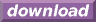#Calcute: freeware calculator

## Natural Logarithm (ln)

The natural logarithm of a number is its logarithm in base e (Euler's number, aka Napier's constant). For a number x, the natural logarithm of x is the power to which e must be raised in order to produce x. Calcute implements the natural logarithm function with ln. The function inverses the effect of raising a number to the power of e so that for some number x, ln(ex) = x.

```ln(2)
0.69314718056
```

Function ln is only defined for numbers greater than zero.